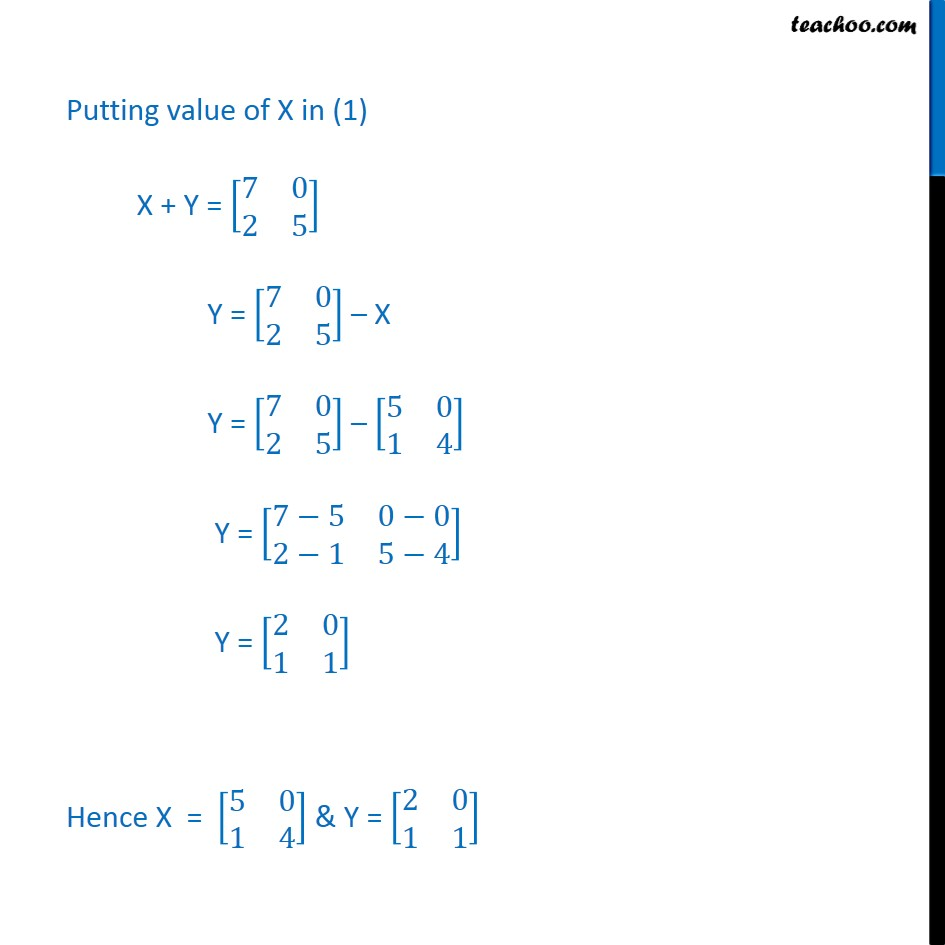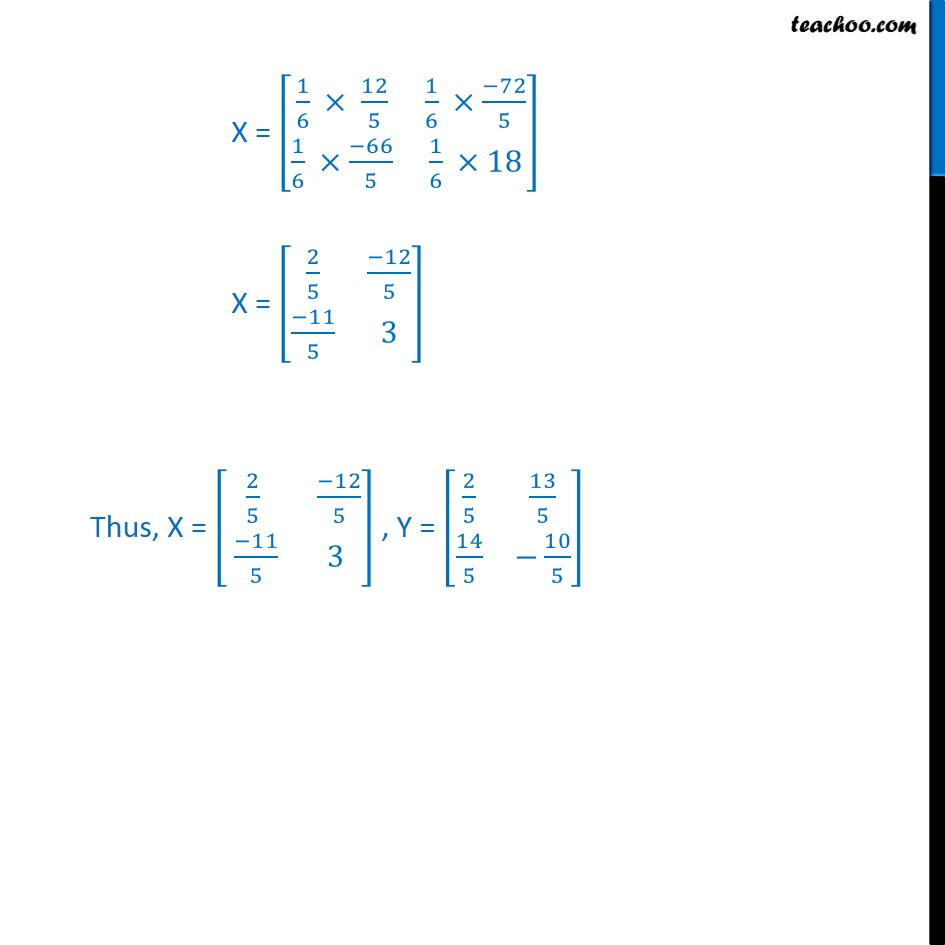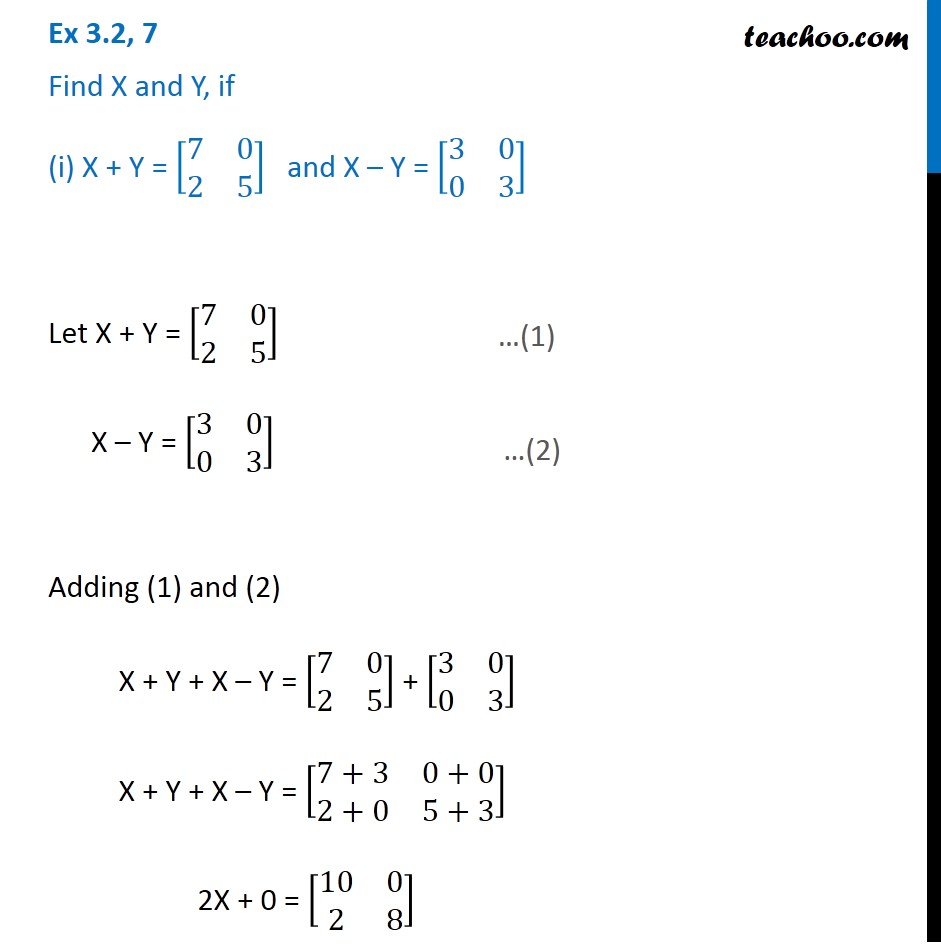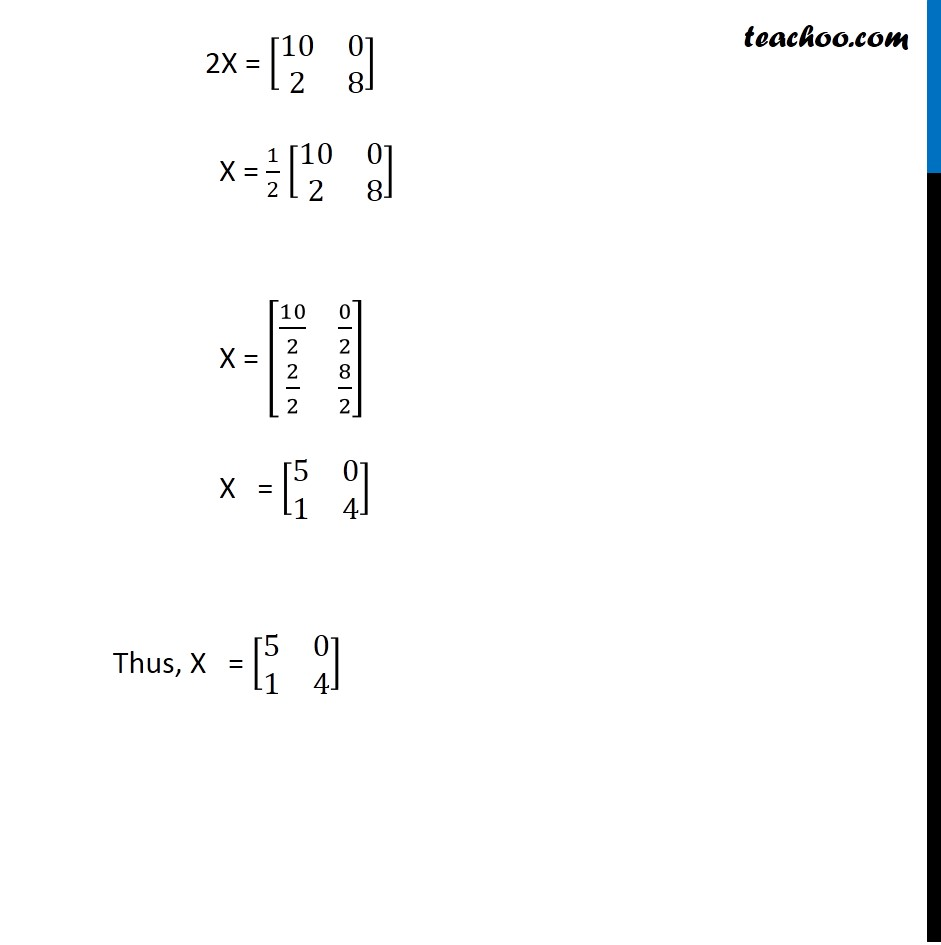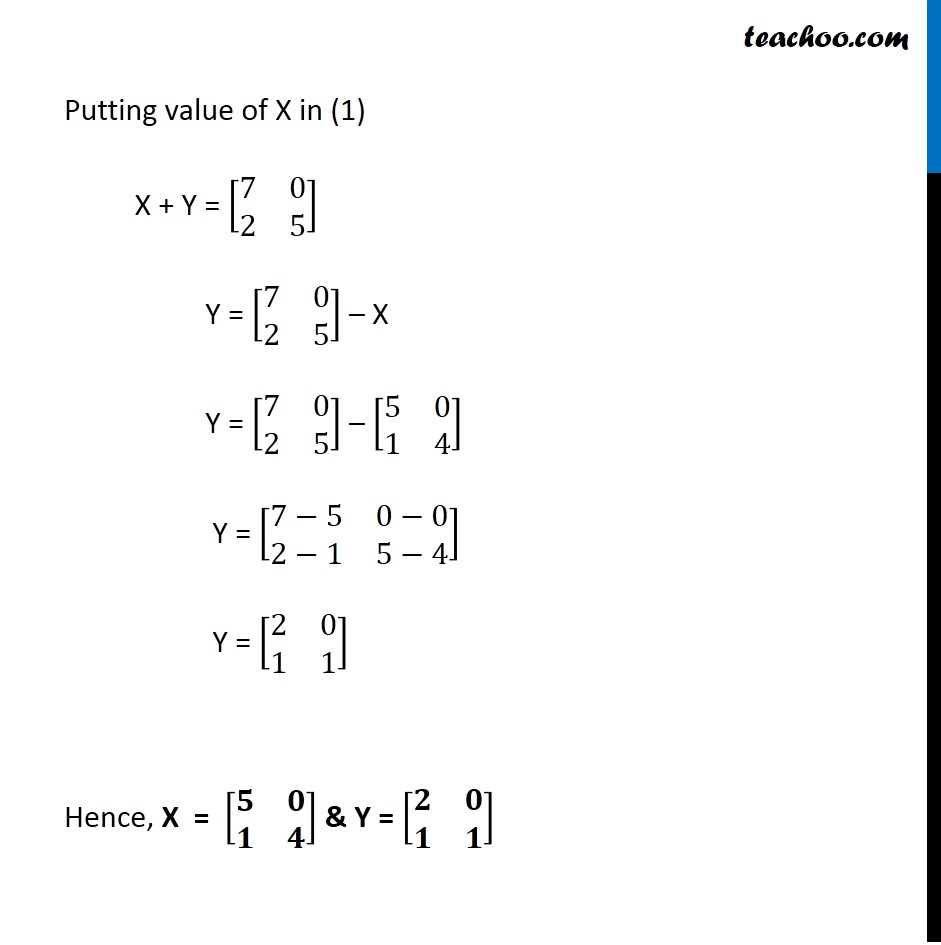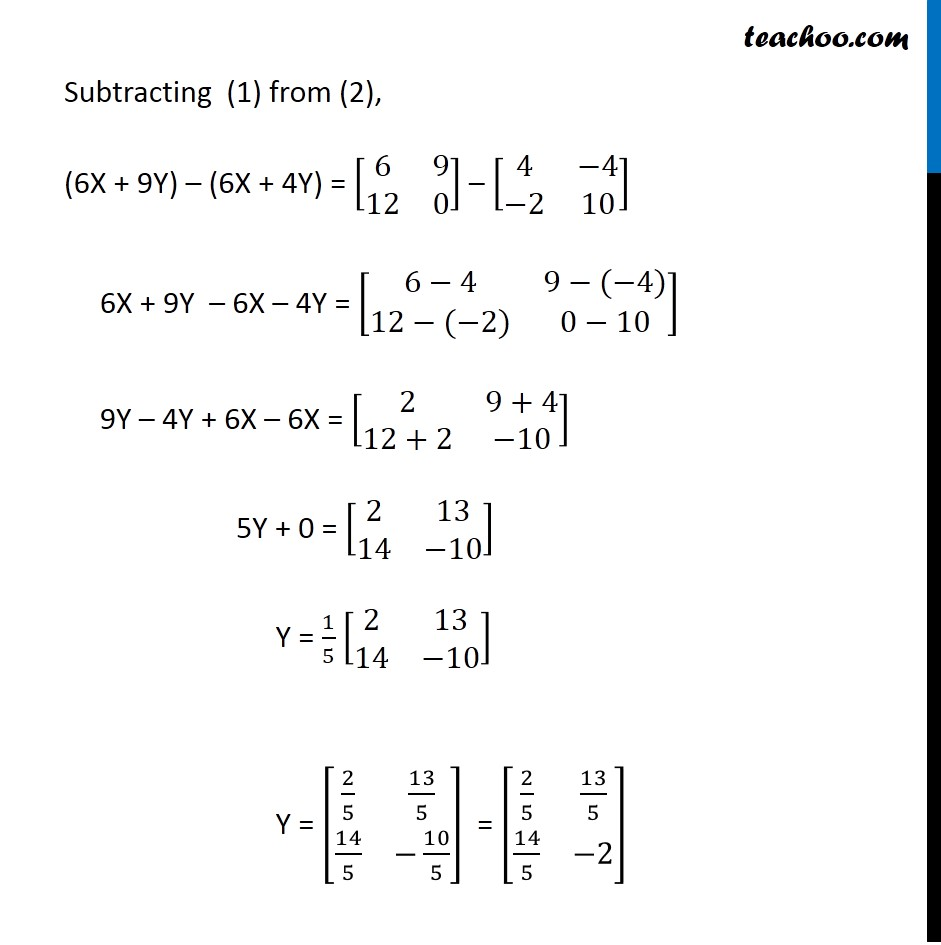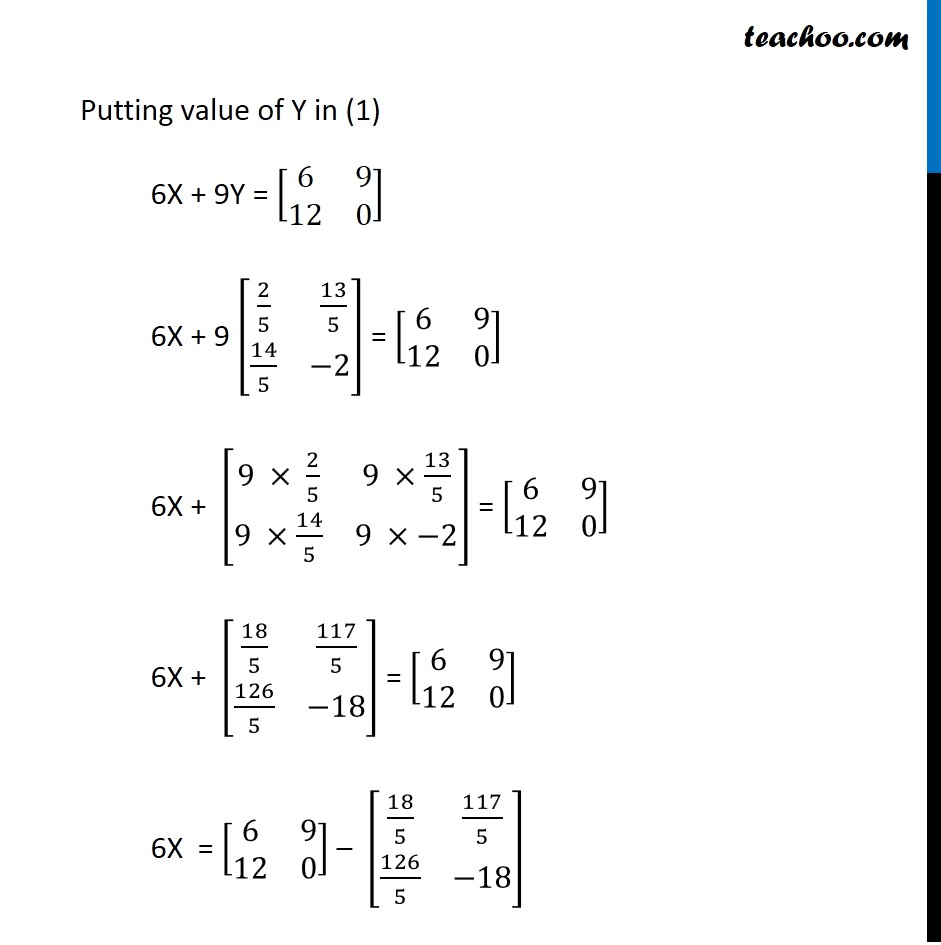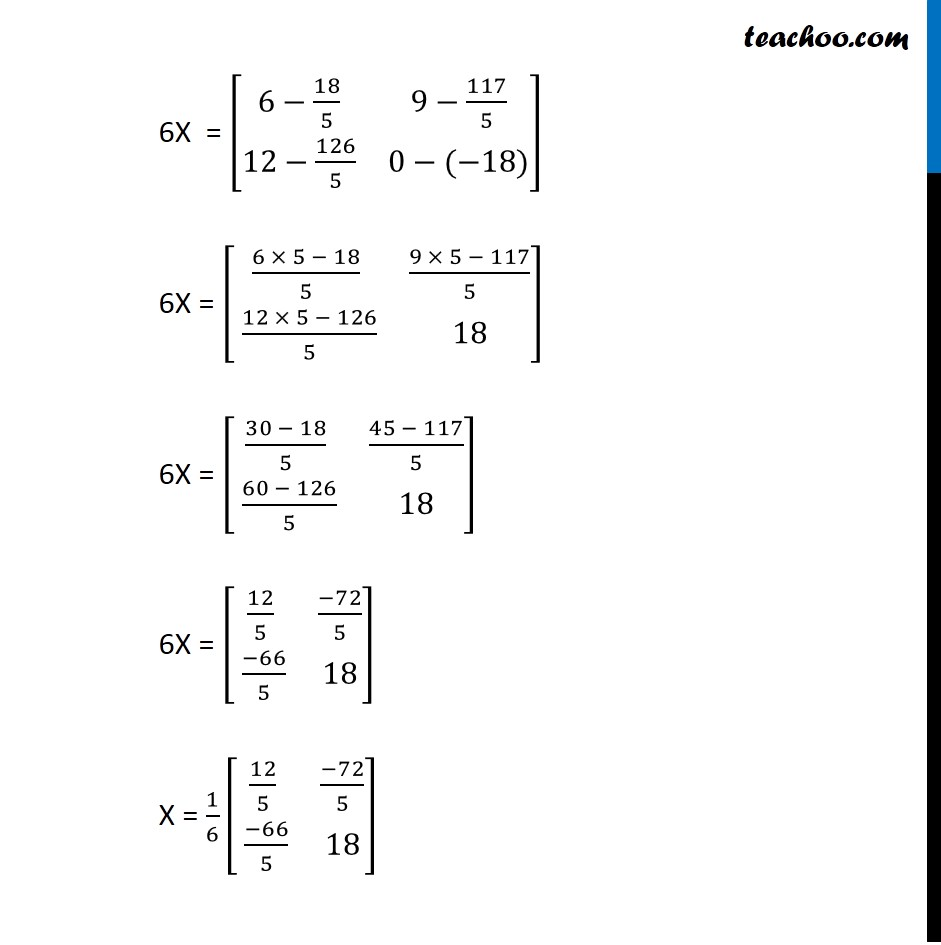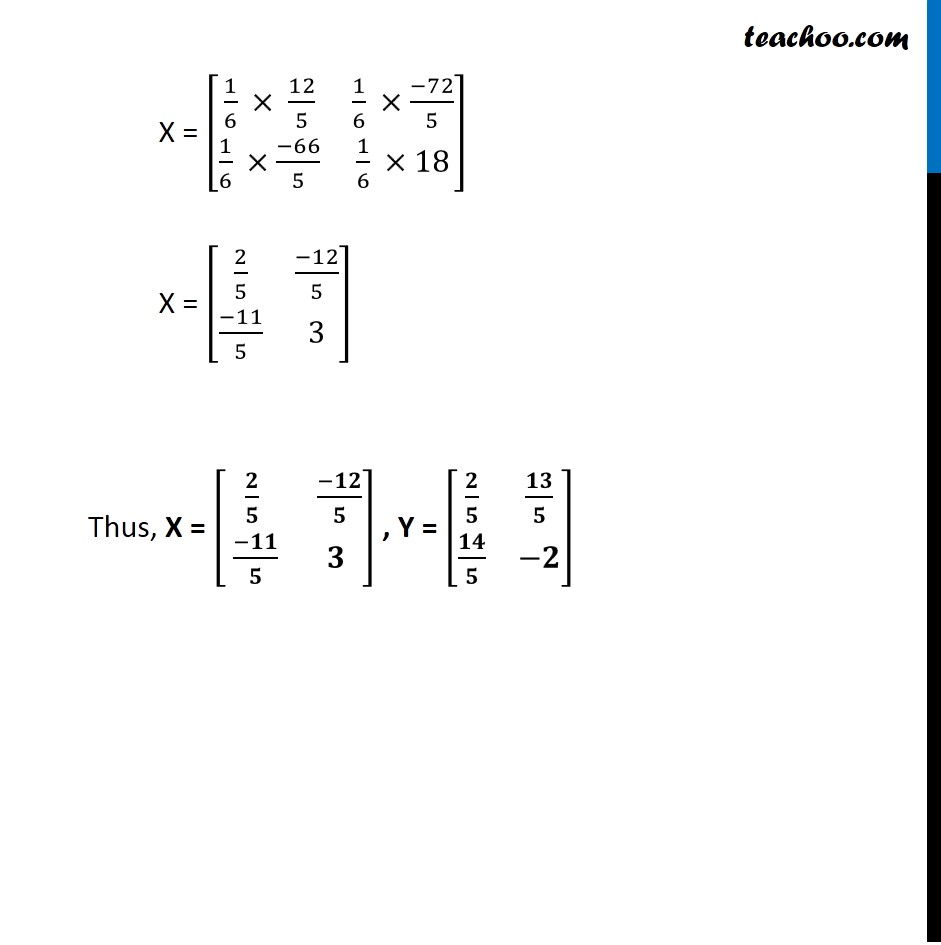1. Chapter 3 Class 12 Matrices
2. Serial order wise
3. Ex 3.2

Transcript

Ex 3.2, 7 Find X and Y, if (i) X + Y = [■8(7&0@2&5)] and X – Y = [■8(3&0@0&3)] Let X + Y = [■8(7&0@2&5)] X – Y = [■8(3&0@0&3)] Adding (1) and (2) X + Y + X – Y = [■8(7&0@2&5)] + [■8(3&0@0&3)] X + Y + X – Y = [■8(7+3&0+0@2+0&5+3)] 2X + 0 = [■8(10&0@2&8)] …(1) …(2) 2X = [■8(10&0@2&8)] X = 1/2 [■8(10&0@2&8)] X = [■8(10/2&0/2@2/2&8/2)] X = [■8(5&0@1&4)] Thus, X = [■8(5&0@1&4)] Putting value of X in (1) X + Y = [■8(7&0@2&5)] Y = [■8(7&0@2&5)] – X Y = [■8(7&0@2&5)] – [■8(5&0@1&4)] Y = [■8(7−5&0−0@2−1&5−4)] Y = [■8(2&0@1&1)] Hence, X = [■8(𝟓&𝟎@𝟏&𝟒)] & Y = [■8(𝟐&𝟎@𝟏&𝟏)] Ex 3.2, 7 Find X and Y, if (ii) 2X + 3Y = [■8(2&3@4&0)] and 3X + 2Y = [■8(2&−2@−1&5)] Given 2X + 3Y = [■8(2&3@4&0)] Multiplying by 3 3 × (2X+ 3Y) = 3 [■8(2&3@4&0)] 6X + 9Y = [■8(2 × 3&3 × 3@4 × 3&0 × 3)] 6X + 9Y = [■8(6&9@12&0)] Given 3X + 2Y = [■8(2&−2@−1&5)] Multiplying by 2 2 × (3X + 2Y) = 2 × [■8(2&−2@−1&5)] 6X + 4Y = [■8(2 ×2&−2 ×2@−1 ×2&5 ×2)] 6X + 4Y = [■8(4&−4@−2&10)] Subtracting (1) from (2), (6X + 9Y) – (6X + 4Y) = [■8(6&9@12&0)] – [■8(4&−4@−2&10)] 6X + 9Y – 6X – 4Y = [■8(6−4&9−(−4)@12−(−2)&0−10)] 9Y – 4Y + 6X – 6X = [■8(2&9+4@12+2&−10)] 5Y + 0 = [■8(2&13@14&−10)] Y = 1/5 [■8(2&13@14&−10)] Y = [■8(2/5&13/5@14/5&−10/5)] = [■8(2/5&13/5@14/5&−2)] Putting value of Y in (1) 6X + 9Y = [■8(6&9@12&0)] 6X + 9 [■8(2/5& 13/5@14/5&−2)] = [■8(6&9@12&0)] 6X + [■8(9 × 2/5&9 ×13/5@9 ×14/5&9 ×−2)] = [■8(6&9@12&0)] 6X + [■8(18/5&117/5@126/5&−18)] = [■8(6&9@12&0)] 6X = [■8(6&9@12&0)] – [■8(18/5&117/5@126/5&−18)] 6X = [■8(6−18/5&9−117/5@12−126/5&0−(−18))] 6X = [■8((6 × 5 − 18)/5&(9 × 5 − 117)/5@ (12 × 5 − 126)/5&18)] 6X = [■8((30 − 18)/5&(45 − 117)/5@ (60 − 126)/5&18)] 6X = [■8(12/5&(−72)/5@ (−66)/5&18)] X = 1/6 [■8(12/5&(−72)/5@ (−66)/5&18)] X = [■8(1/6 × 12/5&1/6 ×(−72)/5@1/6 ×(−66)/5&1/6 ×18)] X = [■8(2/5&(−12)/5@(−11)/5&3)] Thus, X = [■8(𝟐/𝟓& (−𝟏𝟐)/𝟓@ (−𝟏𝟏)/𝟓&𝟑)] , Y = [■8(𝟐/𝟓&𝟏𝟑/𝟓@𝟏𝟒/𝟓&−𝟐)]

Ex 3.2

Chapter 3 Class 12 Matrices
Serial order wise